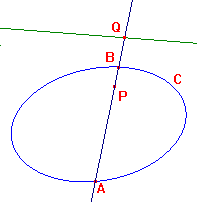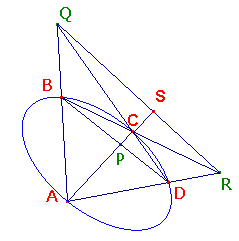some examples involving harmonic pencils

 Cross-ratios of -1 arise quite frequently, For example, we have The polar-chord theorem If a p-point P lies on the chord AB of a p-conic C, then the polar of P with respect to C cuts the p-line AB at Q, where (A,B,P,Q) = -1. Note that this means that, for any p-conic through A and B, the polar of will pass through Q. It is also related to a result which relates the theorems of Ceva and Menelaus, as we shall see.In the projective geometry pages, we met the The Inscribed Quadrilateral Theorem If the vertices of a quadrilateral lie on a p-conic, then the line joining the intersections of opposite sides is the polar of the intersection of the lines joining opposite vertices. In the sketch on the right, the p-line QR is the polar of P. Suppose that the chord AC meets QR at S. Then the above polar-chord theorem shows that (A,C,P,S) = -1. As always, the picture shows an embedding of the projective figure - one in which none of the p-points embeds as an ideal point. In the embedding, we can interpret the cross-ratio in terms of signed ratios (see the embedding theorem). Thus (AP/PC)/(AS/SC) = -1, so that AP/PC = - AS/SC. We can obtain this last equality in another way : Applying Ceva's Theorem to ΔABC and the point D, we get (AP/PC)(CR/RB)(BQ/QA) = 1. Applying Menelaus's Theorem to ΔABC, cut by the line QR, we get (AS/SC)(CR/RB)(BQ/QA) = -1. Comparing these products AP/PC = - AS/SC. From projective geometry, we know that AP/PC = - AS/SC, so either of the theorems of Ceva and Menelaus implies the other. We call this The Ceva-Menelaus Theorem The theorems of Ceva and Menelaus are logically equivalent. In fact, it is easy to show that Menelaus's Theorem implies Ceva's. This was done, in hyperbolic geometry, to prove Ceva's Theorem. The proof in euclidean (or affine) geometry is identical in structure. I know of no way to prove the reverse implication except by using projective geoemtry, or at least harmonic pencils.The polar-chord theorem can also be applied to prove interesting results on plane conics.